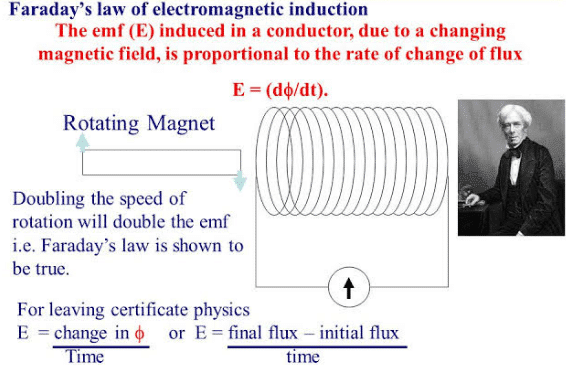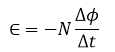Electricity & Megnetism

# Faraday’s law of induction: Definition, Formula and ApplicationsFaraday’s law of induction was discovered through experiments carried out by Micheal Faraday in England In 1831 and by Joseph Henry in the United States at about the same time.
Even though Faraday published his results first, which gives him priority of discovery, the SI unit of inductance is called the henry (abbreviation H). On the other hand, the SI unit of capacitance is, as we have seen, called the farad (abbreviation F).
In the chapter, we discuss oscillations in capacitative-inductive circuits, we see how appropriate it is to link the names of these two talented contemporaries in a single context.

*In addition to their independent simultaneous discovery of the law of induction, Faraday and Henry have several other similarities in their lives. Both were apprentices at an early age. Faraday, at age 14, was apprenticed to a London bookbinder. Henry at age 13, was apprenticed to a watchmaker in Albany, New York. In later years Faraday was appointed a director of the royal institution in London, whose founding was due in large part on an American, Benjamin Thomson (Count Rumford). Henry, on the other hand, because the secretary of the Smithsonian Institution in Washington, DC, which was found by an endowment from an Englishman, James Smithson.

Faraday observed that if a magnet is moved towards a coil of wire (solenoid) connected in series with a galvanometer, an electric current is produced in the current. When the magnet is moved towards the solenoid, the galvanometer shows deflection in one direction and when the magnet is moved away from the solenoid, the galvanometer shows deflection in the opposite direction. When the magnet is stationary there is no deflection in the galvanometer. Similar results are obtained when the magnet is kept stationary and the coil is moved. When the magnet is moved if the deflection in the galvanometer is large and when it is moved slowly the deflection is small. It was also found that if there are two closed circuits in close proximity, one containing a battery and the other a galvanometer, and the battery circuit is closed by pressing the tapping key K, and then broken, the galvanometer in the secondary circuit shows a deflection first in one direction and then in the other.

It is observed that no deflection is produced in the galvanometer if the current in the primary circuit flows continuously. The deflection is produced in the galvanometer only at make or break of the current in the primary circuit. Faraday summed up these experimental results in the form of the following laws:

• 1:Whenever there is a change in the magnetic lines of force or magnetic flux, an induced current is produced in the circuit.
• 2:The induced current or EMF lasts only for the time for which lines of force or magnetic flux is actually changing.
• 3:The magnitude of the induced EMF depends upon the rate at which the magnetic lines of force or magnetic flux changes.

Figure (1) shows a coil of wire as a part of a circuit containing an ammeter. Normally, we would expect the ammeter to show no current in the circuit because there seems to be no electromotive force. However, if we push a bar magnet toward the coil, with its north pole facing the coil, a remarkable thing happens. While the magnet is moving, the ammeter deflects, showing that the current has been set up in the coil. If we hold the magnet stationary with respect to the coil, the ammeter does not deflect. If we move the magnet away from the coil, the meter again deflects, but in the opposite direction, which means that the current in the coil is in the opposite direction. If we use the north pole end of a magnet instead of the north pole end, the experiment works as described but the deflections are reversed. The faster the magnet is moved, the greater is the reading of the meter. Further experimentation shows that what matters is the relative motion of the magnet and the coil. It marks no difference whether we move the magnet toward the coil or the coil toward the magnet.

## Faraday’s law of induction formula

“The induced emf in the circuit is equal to the negative of the rate at which the magnetic flux through the circuit is changing with time.”Mathematically it is written as:Explanation:

Faraday’s experiment showed, and as Faraday’s technique of field lines helps us visualize, it is the change in the number of field lines passing through a circuit loop that induces the emf in the loop. Especially, it is the rate of change in the number of field lines passing through the loop that determines the induced emf.

To make this statement quantitative, we introduce the magnetic flux ΦB, which is  stated as “The number of magnetic lines of force passing normally through the certain area is called magnetic flux.” It is denoted as ΦB. It is a scalar quantity and its SI unit is Weber (Wb). It is measured by the product of magnetic field strength and the component of the vector area parallel to the magnetic field. Mathematically it is represented as:

Φ= B.A

ΦB=BA cosθ

Where A is a vector whose magnitude is the area of the element and whose direction is along the normal to the surface of the element,θ is the angle between the directions of the vectors B and A.

When a magnet is moved toward the loop, the ammeter needle deflects in one direction, as shown in figure (a). When the magnet is brought to rest and held stationary relative to the loop figure (b), no deflection is observed. When the magnet is moved away from the loop, the needle deflects in the opposite direction, as shown in figure (c). Finally, if the magnet is held stationary and the loop is moved either toward or away from it, the needle deflects. From these observations, we conclude that the loop detects that the magnet is moving relative to it and we relate this detection to a change in the magnetic field. Thus, it seems that a relationship exists between the current and changing magnetic fields.

These results are quite remarkable in view of the fact that a current is set up even though no batteries are present in the circuit. We call such a current induced current which is produced by induced emf. This phenomenon is called electromagnetic induction.

There are other related topics on our website are:
1: Lenz’s law
2:Electromagnetic induction
3:Transformer
4:Magnetism
External sources

### Related Articles

1. In general, “ science ” means any body of knowledge systematically ordered in order to describe realities and provide answers to various questions.
The evolution of science is perhaps the most significant development of the human being as a species since throughout the existence of man science progressed substantially.

2. Moosa says:

Very useful. Thanks for all your kindness

3. Michael S. La Moreaux says:

When the magnetic flux linking the circuit changes due to movement or change of size of the circuit, the resultant emf is the motional emf due to the motion of the conductor of the circuit moving through the magnetic field, not the change of flux. Faraday’s Law implies that the emf is due to the change of flux and is therefore not a law. It is not even physics, just math.

4. Michael S. La Moreaux says:

When the magnetic flux linking the circuit changes due to movement or change in size of the circuit, the resultant emf is the motional emf due to the movement of the conductor moving through the magnetic field, not the change in flux. Faraday’s Law implies that the emf is due to the change of flux and is therefore not a law. It is not even physics, just math.